> 文档中心 > 【java刷算法】牛客—剑指offer3栈、数组、递归、二分法的初步练习

# 【java刷算法】牛客—剑指offer3栈、数组、递归、二分法的初步练习

• 🧛‍♂️个人主页：杯咖啡
• 💡进步是今天的活动，明天的保证！
• ✨目前正在学习：SSM框架,算法刷题
• 🙌牛客网，刷算法过面试的神级网站，用牛客你也牛。 👉免费注册和我一起学习刷题👈
• 🐳希望大家多多支持🥰一起进步呀！
• 😎The man who fears losing has already lost.
怕输的人已经输了。 - 《权力的游戏》

# ✨今日三剑

JZ9 用两个栈实现队列
JZ10 斐波那契数列
JZ11 旋转数组的最小数字

### 文章目录

• ✨今日三剑
• JZ9 用两个栈实现队列
• 题目描述
• 思路详解
• 代码与结果
• JZ10 斐波那契数列
• 题目描述
• 思路详解
• 代码与结果
• JZ11 旋转数组的最小数字
• 题目描述
• 思路详解
• 代码与结果
• ✨总结

# JZ9 用两个栈实现队列

## 题目描述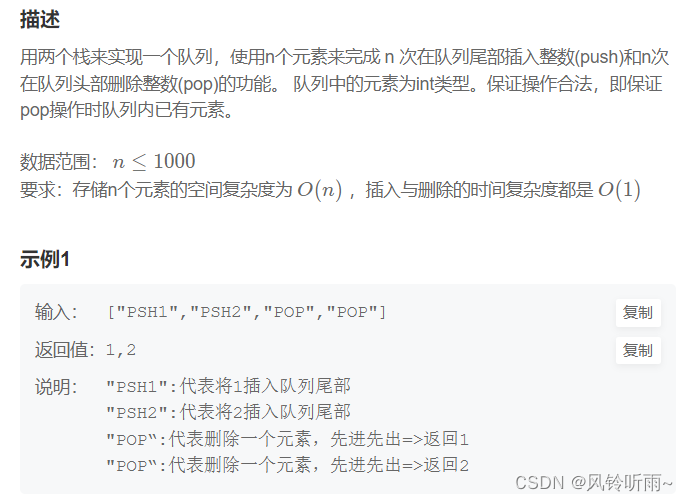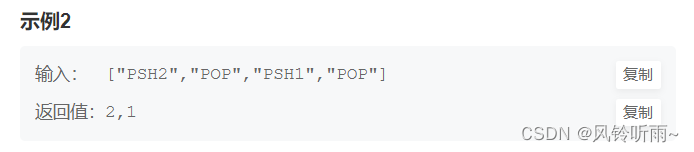## 代码与结果

import java.util.*;import java.util.Stack;public class Solution {    Stack<Integer> stack1 = new Stack<Integer>();    Stack<Integer> stack2 = new Stack<Integer>(); public void push(int node) { stack1.push(node);    } public int pop() { if(stack2.size() <= 0){     while(stack1.size() != 0){  stack2.push(stack1.pop());     } } return stack2.pop();    }}# JZ10 斐波那契数列

## 题目描述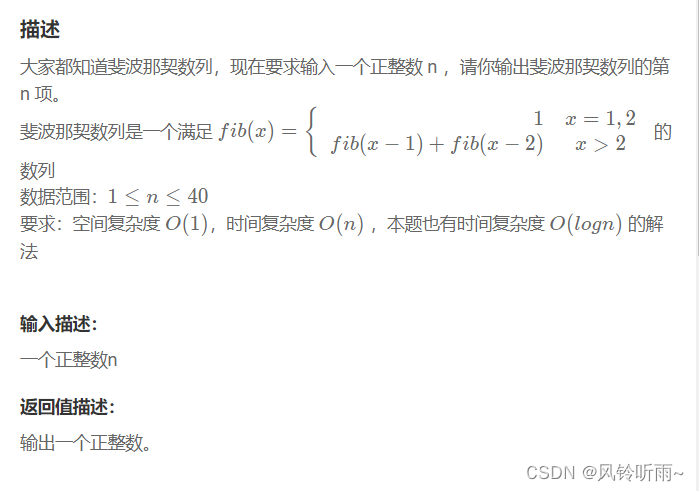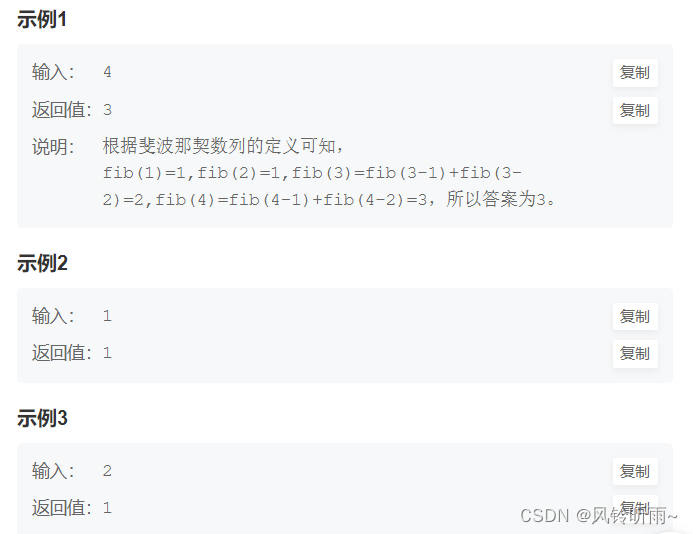## 代码与结果

public class Solution {    public int Fibonacci(int n) { if(n == 1 || n == 2) return 1; else if(n > 2)     return Fibonacci(n - 1) + Fibonacci(n - 2); return 0;    }}# JZ11 旋转数组的最小数字

## 题目描述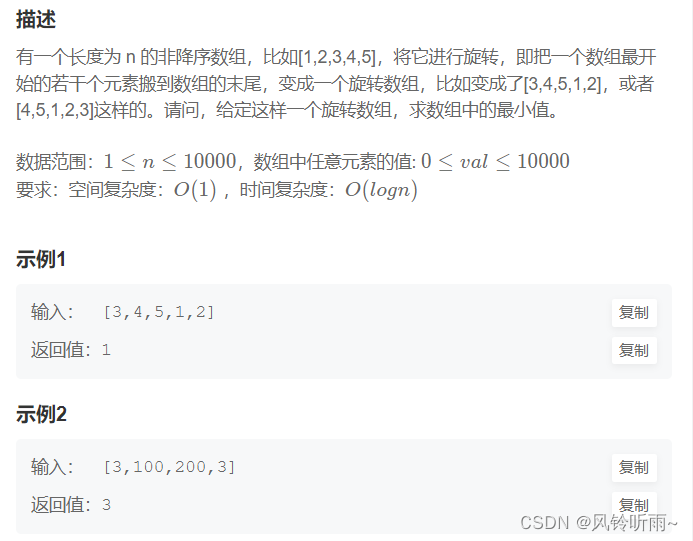## 代码与结果

import java.util.*;import java.util.ArrayList;public class Solution {    public int minNumberInRotateArray(int [] array) {   // 特殊情况判断 if (array.length== 0) {     return 0; } // 左右指针i j int i = 0, j = array.length - 1; // 循环 while (i < j) {     // 找到数组的中点 m     int m = (i + j) / 2;     // m在左排序数组中，旋转点在 [m+1, j] 中     if (array[m] > array[j]) i = m + 1;     // m 在右排序数组中，旋转点在 [i, m]中     else if (array[m] < array[j]) j = m;     // 缩小范围继续判断     else j--; } // 返回旋转点 return array[i];    }}